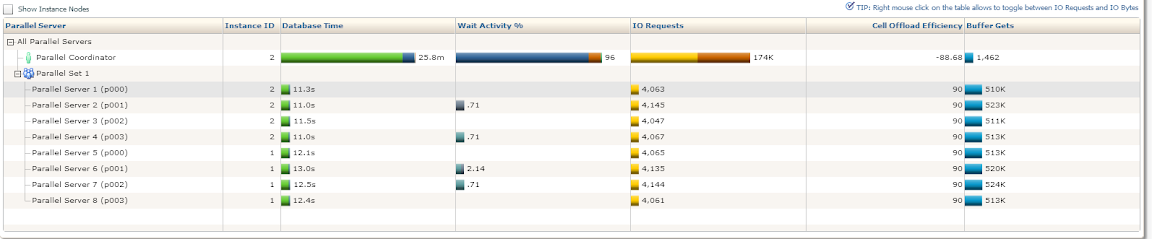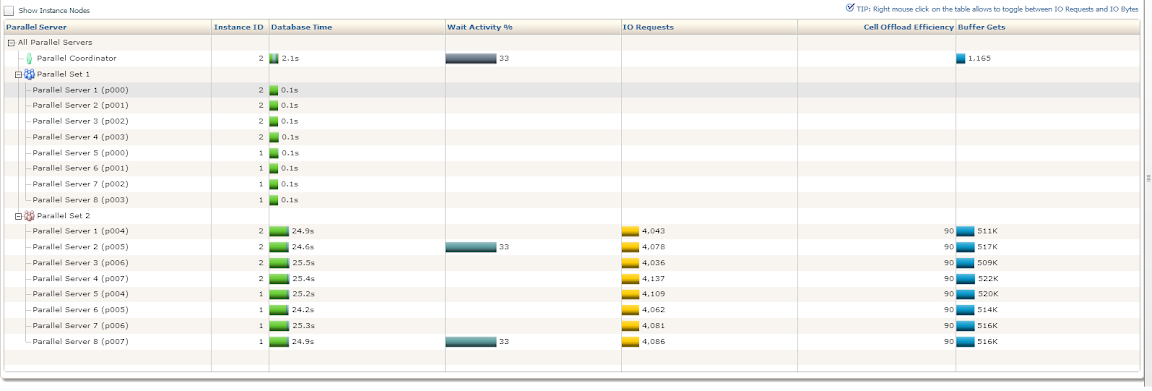## Wednesday, March 13, 2013

### Parallel unfriendly

Take a look at the following Parallel section of a SQL Monitor report:Any query which produces such a report won't care about how much parallel you're running because virtually all the time is spent by the query coordinator (which is a serial process) being busy.

In this case the query in question is quite simple:
`select /*+ parallel(t,8) */ median(basket_amount) from whs.fact_sale t`
The reason it behaves the way it does has everything to do with how Oracle executes it:
```Execution Plan
----------------------------------------------------------
Plan hash value: 712547042

-----------------------------------------------------------------------------------------------------------------------------------------
| Id  | Operation                     | Name       | Rows  | Bytes | Cost (%CPU)| Time     | Pstart| Pstop |    TQ  |IN-OUT| PQ Distrib |
-----------------------------------------------------------------------------------------------------------------------------------------
|   0 | SELECT STATEMENT              |            |     1 |     4 |   110K  (3)| 00:00:03 |       |       |        |      |            |
|   1 |  SORT GROUP BY                |            |     1 |     4 |            |          |       |       |        |      |            |
|   2 |   PX COORDINATOR              |            |       |       |            |          |       |       |        |      |            |
|   3 |    PX SEND QC (RANDOM)        | :TQ10000   |   724M|  2763M|   110K  (3)| 00:00:03 |       |       |  Q1,00 | P->S | QC (RAND)  |
|   4 |     PX BLOCK ITERATOR         |            |   724M|  2763M|   110K  (3)| 00:00:03 |     1 |1048575|  Q1,00 | PCWC |            |
|   5 |      TABLE ACCESS STORAGE FULL| FACT_SALE  |   724M|  2763M|   110K  (3)| 00:00:03 |     1 |1048575|  Q1,00 | PCWP |            |
-----------------------------------------------------------------------------------------------------------------------------------------```
Each parallel query slave will gets it's own chunk of the table to read from and then simply send data back to the coordinator. The coordinator will then have to deal with all this data by sorting more than 700M rows which, of course, won't be particularly fast. In this sense median poses an interesting problem since Oracle can't calculate (or, rather, discover) it without having access to the entire data set and query coordinator is the only process which can do it.

So what do you do when you get impacted by a particular choice of algorithm implemented by Oracle? One way to deal with it is to see whether you can trade one set of problem for another, in case the alternative can be executed in a better way. In this particular case the fact table contains the sale transactions for a particular store chain. While there are many different ways to spent money, the number of distinct spending amounts should be relatively low compared to the number of rows we have in the table and in such a case we can calculate the median in a different way.

What we can do instead is count how many occurrences of each spending we have and, when sorted by the spending amount, that will give us a compressed form of the raw data which still retains all the information required to find a median. Let's say you have a table with the following data:
```SQL> select n from z_t;

N
----------
1
1
2
3
3
5
7

7 rows selected```
The first step is to find how many occurrences of each value do we have:
```SQL> select n, count(*) cnt
2   from z_t
3   group by n;

N        CNT
---------- ----------
1          2
2          1
5          1
3          2
7          1```
If the number of distinct values is relatively low, the group by will be able to collapse the result set enough as to make subsequent work to be not very significant as well as do it in a very parallel friendly way. The next step is to calculate the cardinality of the data set, at which places we have each distinct value as well as how many values are there:
```SQL> select n, lag(running_sum, 1, 0) over (order by n) prev_running_sum, running_sum, total_row_count
2  from (
3  select n,
4   sum(cnt) over (order by n) running_sum,
5   sum(cnt) over () total_row_count
6  from (
7  select n, count(*) cnt
8   from z_t
9   group by n
10  ));

N PREV_RUNNING_SUM RUNNING_SUM TOTAL_ROW_COUNT
---------- ---------------- ----------- ---------------
1                0           2               7
2                2           3               7
3                3           5               7
5                5           6               7
7                6           7               7```
So what the above tells us is we have two 1s, followed by a single 2, followed by two 3s and so on. Because we have seven elements in our data set, we know that the median will be the item number four which we can now easily discover:
```SQL> select avg(n) from (
2  select n, lag(value_begin, 1, 0) over (order by n) prev_value_begin, value_begin, total_row_count
3  from (
4  select n,
5   sum(cnt) over (order by n) value_begin,
6   sum(cnt) over () total_row_count
7  from (
8  select n, count(*) cnt
9   from z_t
10   group by n
11  ))) where total_row_count/2 between prev_value_begin and value_begin;

AVG(N)
----------
3```
The avg is there for a case where we have an even number of elements since the median in this case equals to a mean value of two values in the middle.

Our new real query will look like this:
```select avg(n) from (
select n, lag(value_begin, 1, 0) over (order by n) prev_value_begin, value_begin, total_row_count
from (
select n,
sum(cnt) over (order by n) value_begin,
sum(cnt) over () total_row_count
from (
select /*+ parallel(t,8) */ basket_amount n, count(*) cnt
from whs.fact_sale tThe total execution time has dropped from almost 26 minutes down to 28 seconds. Moreover, the workload is now much more skewed towards parallel query slaves, which is exactly what we want to see. Of course, the trick only works if the group by is able to collapse the data sufficiently enough.